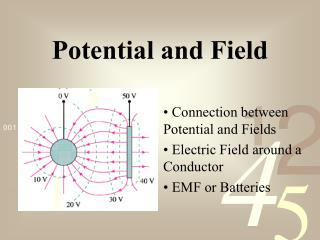DownloadDownload PresentationPotential and Field

# Potential and Field

Download Presentation## Potential and Field

- - - - - - - - - - - - - - - - - - - - - - - - - - - E N D - - - - - - - - - - - - - - - - - - - - - - - - - - -
##### Presentation Transcript

1. Potential and Field Connection between Potential and Fields Electric Field around a Conductor EMF or Batteries

2. Reading Questions What quantity is represented by the symbol ? 1. Electronic potential 2. Excitation potential 3. Electromotive force 4. Electric stopping power 5. Exosphericity

3. Reading Questions What quantity is represented by the symbol ? 1. Electronic potential 2. Excitation potential 3. Electromotive force 4. Electric stopping power 5. Exosphericity

4. Reading Questions The electric field 1. is always perpendicular to an equipotential surface. 2. is always tangent to an equipotential surface. 3. always bisects an equipotential surface. 4. makes an angle to an equipotential surface that depends on the amount of charge.

5. Reading Questions The electric field 1. is always perpendicular to an equipotential surface. 2. is always tangent to an equipotential surface. 3. always bisects an equipotential surface. 4. makes an angle to an equipotential surface that depends on the amount of charge.

6. Reading Questions The relationship ∆V=IR is called 1. Faraday’s law. 2. Ampere’s law. 3. Ohm’s law. 4. Maxwell’s equation. 5. No name was given in this chapter.

7. Reading Questions The relationship ∆V=IR is called 1. Faraday’s law. 2. Ampere’s law. 3. Ohm’s law. 4. Maxwell’s equation. 5. No name was given in this chapter.

8. Energy concept Electric Field and Potential Force concept Acts locally Everywhere in space

9. Hand waving argument using a constant electric field Connecting Potential and Field Recall from the last lecture we found From Calculus III we find

10. Energy concept Electric Field and Potential Force concept Acts locally Everywhere in space

11. TACTICS BOX 30.1 ‑Finding the potential difference from the electric field • 1) Draw a picture and identify the two points between which you wish to find the potential. Call them positions i and f. • If you need to assign a specific value of V to a point in space, choose position f to be the zero point of the potential, usually at infinity. • Establish a coordinate axis from i to f along which you already know or can easily determine the electric field component • 4) Carry out the integral of Equation 30.3 to find the potential difference Potential from Electric Field Calculating the potential from the electric field.

12. Or for one dimension Electric Field from Potential Recall

13. Electric Field and Potential

14. Electric Field from Potential

15. Electric Field and Potential Kirchhoff’s Loop Law The sum of the voltages aroung a closed path equal zero.

16. Student Workbook

17. Student Workbook You should do this one.

18. Vector so - - Student Workbook You should do this one.

19. Student Workbook

20. Student Workbook

21. Student Workbook

22. Student Workbook

23. Class Question Which potential-energy graph describes this electric field? 1. a 2. b 3. c 4. d 5. e

24. Class Question Which potential-energy graph describes this electric field? 1. a 2. b 3. c 4. d 5. e

25. Which set of equipotential surfaces matches this electric field? (1) (2) (3) (5) (4) Class Question

26. Which set of equipotential surfaces matches this electric field? (1) (2) (3) (5) (4) Class Question

27. Gaussian surface Electric Field around Conductors + +

28. Electric Field and Potential

29. Electric Field and Potential

30. Student Workbook

31. Student Workbook

32. Student Workbook

33. Class Question Three charged, metal spheres of different radii are connected by a thin metal wire. The potential and electric field at the surface of each sphere are V and E. Which of the following is true? 1. V1 = V2 = V3 and E1 = E2 = E3 2. V1 = V2 = V3 and E1 > E2 > E3 3. V1 > V2 > V3 and E1 = E2 = E3 4. V1 > V2 > V3 and E1 > E2 > E3 5. V3 > V2 > V1 and E1 = E2 = E3

34. Class Question Three charged, metal spheres of different radii are connected by a thin metal wire. The potential and electric field at the surface of each sphere are V and E. Which of the following is true? 1. V1 = V2 = V3 and E1 = E2 = E3 2. V1 = V2 = V3 and E1 > E2 > E3 3. V1 > V2 > V3 and E1 = E2 = E3 4. V1 > V2 > V3 and E1 > E2 > E3 5. V3 > V2 > V1 and E1 = E2 = E3

35. EMF or Battery

36. EMF or Battery

37. Student Workbook You should do this one.

38. Student Workbook

39. Class Question A wire connects the positive and negative terminals of a battery. Two identical wires connect the positive and negative terminals of an identical battery. Rank in order, from largest to smallest, the currents Ia to Id at points a to d. 1. Ia =Ib =Ic =Id 2. Ia =Ib >Ic =Id 3.Ic =Id >Ia =Ib 4. Ic =Id >Ia >Ib 5. Ia >Ib >Ic =Id

40. Class Question A wire connects the positive and negative terminals of a battery. Two identical wires connect the positive and negative terminals of an identical battery. Rank in order, from largest to smallest, the currents Ia to Id at points a to d. 1. Ia =Ib =Ic =Id 2. Ia =Ib >Ic =Id 3.Ic =Id >Ia =Ib 4. Ic =Id >Ia >Ib 5. Ia >Ib >Ic =Id